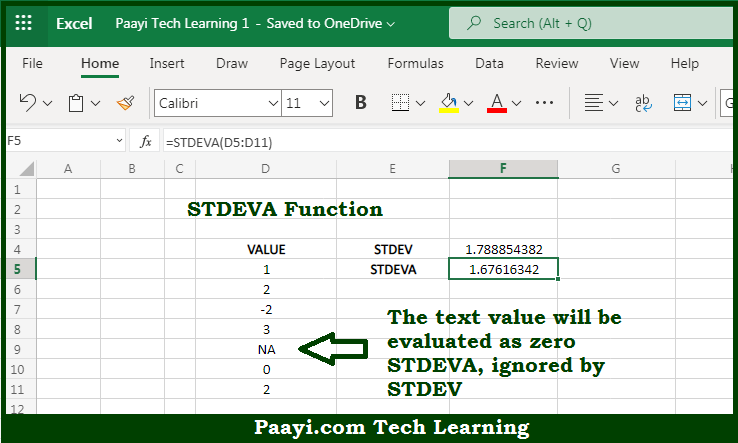# Learn How to Use Microsoft Excel STDEVA Function

Written by | 0 Comments | 556 Views

In this article, you will learn how to use the Microsoft Excel STDEVA function and its prime function in Microsoft Excel. You will also get to know the Microsoft Excel STDEVA function return value and syntax with the help of some examples.

Microsoft Excel STDEVA Function

The main purpose of the Microsoft Excel STDEVA function is to get the standard deviation in the sample. That implies, with the help of the STDEVA function you can able to return the standard deviation for data that represents a sample or population. It should be noted that the STDEVA function will evaluate text and the logical that appear in references, unlike the STDEV function. You can use STDEVPA or STDEV.P, to calculate the standard deviation for an entire population. So, with the help of the STDEVA function, you can able to get the standard deviation in the given sample or population.

Return Value of STDEVA Function

The return value will be the estimated standard deviation.

Syntax of STDEVA Function

=STDEVA(number1, [number2], ...)

Where the arguments:

• number1: This is the first number or reference in the sample.
• number2: This is the second number or reference in the sample (optional).

## How to Use Microsoft Excel STDEVA Function?So we know that Microsoft Excel STDEVA function you can able to get the standard deviation in the sample. That implies, with the help of the STDEVA function you can able to return the standard deviation for data that represents a sample or population. It should be noted that the STDEVA function will evaluate text and the logical that appear in references, unlike the STDEV function. You can use STDEVPA or STDEV.P, to calculate the standard deviation for an entire population,. So, with the help of the STDEVA function, you can able to get the standard deviation in the given sample or population.